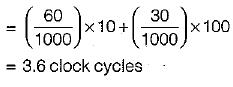Courses

# Cache And Main Memory (Advance Level) - 1

## 15 Questions MCQ Test Question Bank for GATE Computer Science Engineering | Cache And Main Memory (Advance Level) - 1

Description
This mock test of Cache And Main Memory (Advance Level) - 1 for Computer Science Engineering (CSE) helps you for every Computer Science Engineering (CSE) entrance exam. This contains 15 Multiple Choice Questions for Computer Science Engineering (CSE) Cache And Main Memory (Advance Level) - 1 (mcq) to study with solutions a complete question bank. The solved questions answers in this Cache And Main Memory (Advance Level) - 1 quiz give you a good mix of easy questions and tough questions. Computer Science Engineering (CSE) students definitely take this Cache And Main Memory (Advance Level) - 1 exercise for a better result in the exam. You can find other Cache And Main Memory (Advance Level) - 1 extra questions, long questions & short questions for Computer Science Engineering (CSE) on EduRev as well by searching above.
QUESTION: 1

### A system which has lot of crashes, data should be written to the disk using

Solution:

System should write data immediately So called it write-back.

QUESTION: 2

### The average memory access time for a machine with a cache hit rate of 90% where the cache access time is 10 ns and the memory access time is 100 ns is

Solution:

Average memory access time = Hit Ratio x Cache access time + Miss Ratio x Memory access time
= 0.90 x 10 ns + 0.10 x 100 ns
= 9 ns + 10 ns = 19 ns

QUESTION: 3

### Given below are some statements associated with cache memory. Identify the correct statement.

Solution:

The level-1 cache may be of same speed as level- 2 the level-2 cache used to mitigate the dynamic slow down every time a level-1 cache miss occur level-1 cache comes on processor chip while level-2 cache comes on external chip.

QUESTION: 4

Which of the following holds data and processing instructions temporarily until the CPU needs it?

Solution:

All the data and instruction before start executing presents in main memory temporarily.

QUESTION: 5

Suppose a cache is 10 times faster than main memory and suppose that the cache can be used 70% of the time. How much speed up do we gain by using the cache?

Solution:

In Flynn’s classification arrays and vectors belongs to SIMD architecture.

QUESTION: 6

Given below are some statements associated with computer memory. Identify the correct statement.

Solution:

Main memory is used by the processor to store primary active program and data.

QUESTION: 7

In a two level memory hierarchy, the access time of the cache memory is 12 nsec and the access time of the main memory is 1.5 msec. The hit ratio is 0.98. What is the average access time of the two level memory system?

Solution:

Average access time = Hit Ratio x Cache access time + Miss Ratio x Memory access time Here, Memory access time
= 1.5 μs i.e. 1500 ns
So, average access time
= 0.98 x 12 ns + 0.02 x 1500 ns
= 11.76 ns + 30 ns = 41.76 ns

QUESTION: 8

Determine True(T) / False(F) of the following statements.
1. Dynamic RAM consists of internal flip-flops.
2. Static RAM consists of capacitors.
3. Static RAM is easier to use than dynamic RAM.
4. Static RAM has shorter read and write cycles than that of dynamic RAM

Solution:

Static random access memory is a type of semiconductor memory that uses bistable latching circuitry or flip-flop while dynamic random access memory uses capacitor. SRAM is easier to use and has smaller read and write cycles than that of DRAM.

QUESTION: 9

Consider the following organization of main memory and cache memory:
Main memory: 64K x 16
Cache memory; 256 x 16
Memory is word-addressable and block size is of 8 words. Determine the size of tag field if direct mapping is used for transforming data from main memory to cache memory.

Solution:

Number of words in cache = 256 = 28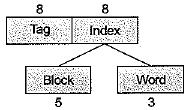QUESTION: 10

A certain computer system design has a single CPU, a two-level cache, and supports memory mapped I/O for output-only controllers. Which of the following is true?

Solution:

In 2-level cache, level-2 cache is generally larger than and equal to level-1 cache, level-1 cache has access detency less than level-2 cache and L1 cache generally build from SRAM.

QUESTION: 11

The designer of a cache system need to reduces the number of cache misses that occur in a certain group of programs. Which of the following statements is/are true?
1. If compulsory misses are most common then the designers should consider increasing the cache line size to take better advantage of locality.
2. If capacity misses are most common then the designer should consider increasing the total cache size so it can contain more lines.
3. If conflict misses are most common then the designers should increasing the cache’s associativity, in order to provide more flexibility when collision occurs.

Solution:

To reduce the number of cache misses:

1. If compulsory misses are common, then increase cache line size will take better advantage of locality i.e. more number of entries will be present in cache at a time.
2. If conflict misses are common then increasing cache associativity will provide more flexibility.
3. If capacity misses are common then increasing total cache size so that maximum entries can be contained in it.

QUESTION: 12

Assume a fully associative write-back cache with many cache entries that starts empty. We define a sequence of memory operations. The address or locations is in square brackets.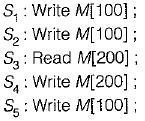Q. What are the number of hits when using no write allocate versus write allocate?

Solution:

For no write allocate, the fourth or write M is a hit so is in cache so only 1 hit. For write allocate S2, S4, S5 are hit so 3 hits.
So pair contains 1,3.

QUESTION: 13

Assume a fully associative write-back cache with many cache entries that starts empty. We define a sequence of memory operations. The address or locations is in square brackets.Q. What are the number of misses when using no write allocate versus write allocate?

Solution:

Number of misses for no write allocate are S1, S2, S3 and S5. For write allocate misses are S1 and S3. So pair contains 4, 2.

QUESTION: 14

Suppose a CPU contains 1000 memory references there are 40 misses in L1 cache (First Level Cache) and 20 misses in the L2 cache (Second Level Cache). Assume miss penalty from the L2 cache to memory is 100 clock cycles the hit time of L2 cache is 10 clock cycles, the hit time of L1 cache is 1 clock cycle and there are 1.5 memory references per instruction.

Q. What is the average memory access time?

Solution: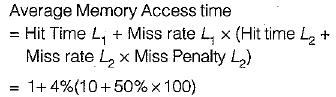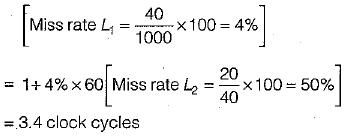QUESTION: 15

Suppose a CPU contains 1000 memory references there are 40 misses in L1 cache (First Level Cache) and 20 misses in the L2 cache (Second Level Cache). Assume miss penalty from the L2 cache to memory is 100 clock cycles the hit time of L2 cache is 10 clock cycles, the hit time of L1 cache is 1 clock cycle and there are 1.5 memory references per instruction.

Q. What is average memory stalls per instruction?

Solution:

Average Memory Stall per instruction

= Misses Per instruction L1 x Hit time L2 + Misses per instruction L2 x Miss penalty L2